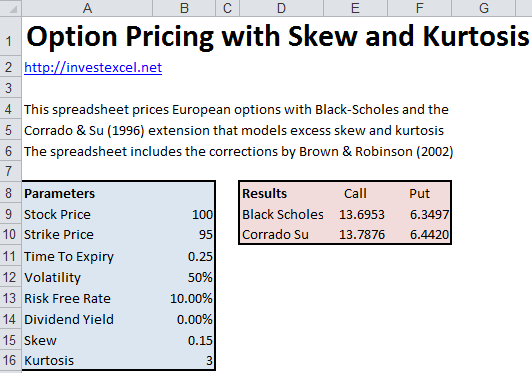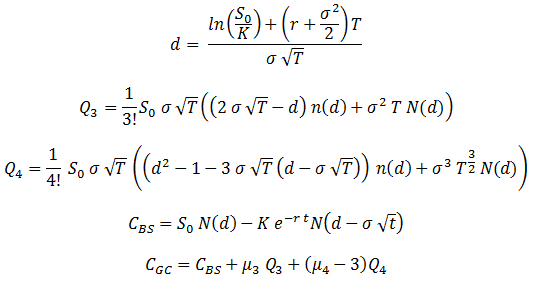# Option Pricing with Skew and Kurtosis

Learn about the Corrado & Su (1996) model for pricing options with excess skew and kurtosis, and get a pricing spreadsheet.

The Black-Scholes option pricing model has several well-known deficiencies. Perhaps most significantly, Black-Scholes assumes that prices are log-normally distributed.

In reality, howeer, investors see more extreme behavior than predicted by the normal distribution; in fact, extreme events occur 10 times more often than the normal distribution would have you assume.Extreme behavior in returns distributions can be described by two statistical quantities known as skew and kurtosis.

Skewed returns distributions are not symmetric. If the returns distribution has

• positive skew, you should expect many smaller negative returns, and a few larger positive returns; your downside-risk is minimized.
• negative skew, you should expect many smaller positive returns, and a few larger negative returns; this can be a real source of worry for risk-averse investors

Kurtosis describes the relative “peakiness” or “flatness” of a returns distribution compared to the normal distribution. A peaky distribution has fat tails, with a lower probability of extreme behavior (this is also known as leptokurtic).

Corrado & Su (1996)  extended the standard Black-Scholes scheme for option pricing by capturing the effect of skew and kurtosis. Their novel approach expanded the normal density function with a Gram-Charlier approach. This resulted in a pricing formula that was equal to the standard Black-Scholes equations plus terms that capture excess skew and kurtosis.

The prices predicted by the Corrado & Su (1996) equations are equal to those predicted by Black-Scholes for a skew of 0 and kurtosis of 3.

Brown and Robinson(2002) corrected an error in the Corrado & Su (1996) equations. The corrected equations arewhere

• K is the strike price
• S0 is the asset price
• r is the risk-free rate
• σ is the volatility
• T is the time to expiry
• CBS is the option price predicted by the Black-Scholes model
• μ3 is the skew
• μ4 is the kurtosis
• n(d) and N(d) are the standard normal density and the standard normal distribution

This Excel spreadsheet prices European options with both the standard Black-Scholes approach, and the Corrado & Su (1996) extension for excess skew and kurtosis (including the Brown & Robinson (2002) correction).

The quations are programmed in VBA, which can be viewed, edited and used in your own applications. Please link to http://investexcel.net if you find the spreadsheet of value.

Download Excel Spreadsheet to Price European Options with Skew and Kurtosis